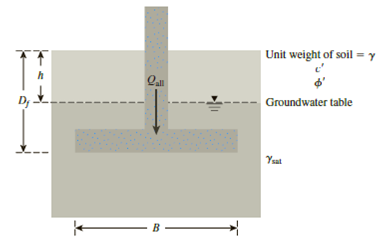Chapter 16, Problem 16.10PPrinciples of Geotechnical Enginee...

9th Edition
Braja M. Das + 1 other
ISBN: 9781305970939

Solutions

Chapter
SectionPrinciples of Geotechnical Enginee...

9th Edition
Braja M. Das + 1 other
ISBN: 9781305970939
Textbook Problem

If the water table in Problem 16.9 drops down to 0.25 m below the foundation level, what would be the change in the factor of safety for the same gross allowable load?16.9 A square footing is shown in Figure 16.18. Determine the gross allowable load, Qall, that the footing can carry. Use Terzaghi’s equation for general shear failure (Fs = 4). Given: γ = 17 kN/m3, γsat = 19.2 kN/m3, c′ = 32 kN/m3, ϕ ′ = 26 ° , Df = 1 m, h = 0.5 m, and B = 1.5 m.Figure 16.18

To determine

Find the change in factor of safety for the estimated gross allowable load.

Explanation

Given information:

The factor of safety Fs is 4.0.

The unit weight of the soil γ is 17kN/m3.

The saturated unit weight of the soil γsat is 19.2kN/m3.

The value of cohesion c is 32kN/m2.

The soil friction angle ϕ is 26°.

The depth of foundation Df is 1.0 m.

The depth of soil above water table h is 0.5 m.

The drop down depth of water table D is 0.25 m.

The width of footing B is 1.5 m.

Calculation:

For depth of water table at 0.5 m.

Determine the magnitude of q using the relation.

q=γ(Dfh)+γh=γ(Dfh)+(γsatγw)h (1)

Here, γ is the effective unit weight of soil and γw is the unit weight of water.

Take unit weight of water as 9.81kN/m3.

Substitute 17kN/m3 for γ, 1.0 m for Df, 0.5 m for h, 19.2kN/m3 for γsat, and 9.81kN/m3 for γw in Equation (1).

q=17(1.00.5)+(19.29.81)0.5=13.19kN/m2

Determine the ultimate bearing capacity of the soil (qu) using the relation.

qu=1.3cNc+qNq+0.4γBNγ

Here, Nc is the contribution of cohesion, Nq is the contribution of surcharge, and Nγ is the contribution of unit weight of soil.

Refer Table 16.1, “Terzaghi’s bearing-capacity factors–Nc, Nq, and Nγ” in the textbook.

Take the Nc as 27.09, Nq as 14.21, and Nγ as 9.84 for the ϕ value of 26°.

Substitute 32kN/m2 for c, 27.09 for Nc, 13.19kN/m2 for q, 14.21 for Nq, (19.29.81)kN/m3 for γ, 1.5 m for B, and 9.84 for Nγ.

qu=1.3×32×27.09+13.19×14.21+0.4×(19.29.81)×1.5×9.84=1,369.81kN/m2

Determine the gross allowable load (qall) that the footing can carry using the relation.

qall=quB2Fs

Substitute 1,369.81kN/m2 for qu, 1.5 m for B, and 4.0 for Fs.

qall=1,369.81×1.524.0=771kN

For drop down depth of ground water table at 0

Still sussing out bartleby?

Check out a sample textbook solution.

See a sample solution

The Solution to Your Study Problems

Bartleby provides explanations to thousands of textbook problems written by our experts, many with advanced degrees!

Get Started

Why is it important to know the Greek alphabet?

Engineering Fundamentals: An Introduction to Engineering (MindTap Course List)

What are the three basic operations in cryptography?

Principles of Information Security (MindTap Course List)

What metals can be cut with the oxyfuel gas process?

Welding: Principles and Applications (MindTap Course List)

Which is faster: sequential or direct access? Why? (392)

Enhanced Discovering Computers 2017 (Shelly Cashman Series) (MindTap Course List)